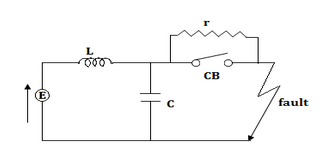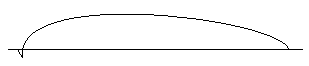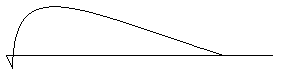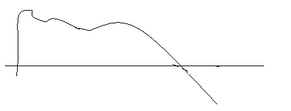# Restriking Voltage in Circuit Breakers - 2 - MCQs with answers

## Restriking Voltage in Circuit Breakers - 2 - MCQs with answers

Q1. How is the restriking voltage measured?

a. RMS value
b. Peak value
c. Instantaneous value
d. Average value

Q2. The making and breaking currents of a 3 phase ac circuit breakers in power systems are respectively in what form?

a. rms value, rms value
b. instantaneous value, rms value
c. rms value, instantaneous value
d. instantaneous value, instantaneous value

ANSWER: d. Instantaneous value, instantaneous value

Q3. In a short circuit test on a circuit breaker, the following readings were obtained on single frequency transients

a. Time to reach the peak re striking voltage - 50μ sec
b. The peak re striking voltage, 100 kV

What is its average RRRV?

a. 2*106kV/sec
b. 3*105kV/sec
c. 2*105kV/sec
d. 3*106kV/sec

Q4. In the above question, for the same data what will be the frequency of oscillations

a. 100 Hz
b. 1,000 Hz
c. 10,000 Hz
d. 10 Hz

Refer to the following facts to answer Question 5 & 6

A 50 Hz, 11 kV, 3 phase alternator with earthed neutral having a reactance of 3 ohms per phase and is connected to a bus bar through a circuit breaker, if the distributed capacitance upto CB between the phase and neutral is 0.01 μ F.

Q5. What is the peak re striking voltage?

a. 18.36 kV
b. 17.96 kV
c. 15.96 kV
d. 12.65 kV

Q6. What is the frequency of oscillations?

a. 10000 Hz
b. 12500 Hz
c. 12628 Hz
d. 13265 Hz

Q7. What is the average rate of rise of restriking voltage upto the first peak?

a. 525 * 103kV / sec
b. 453 * 103kV / sec
c. 582 * 103 kV / sec
d. 467 * 103 kV / sec

ANSWER: b. 453 * 103kV / sec

Q8. A 50 Hz 3 phase synchronous generator has inductance per phase of 15mH. The capacitance of generator and the circuit breaker is 0.002μ F. What is the natural frequency of oscillation?

a. 29 kHz
b. 2.9 kHz
c. 290 kHz
d. 29 MHz

Q9. For the following figure shows a CB circuit with L - C introducing a damping circuit. For the critical damping what is the value of r?a. √(LC)
b. 0.5*√(C/L)
c. 0.5*√(L/C)
d. 2π*√(L/C)

Q10. Which among the following graphs represent the oscillation R < {0.5 √ (L/C)}

a.b.c.d. All of the above.